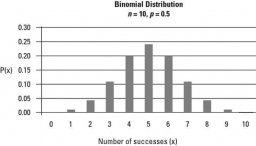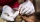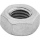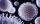# Probability of failures

In certain productions, the probability of failures is 0.01. Calculate the probability that there will be more than 1 failure among the 100 selected products if we return the selected products to the file after the check.

Correct result:

p =  0.2642

#### Solution:We would be pleased if you find an error in the word problem, spelling mistakes, or inaccuracies and send it to us. Thank you!Tips to related online calculators
Looking for a statistical calculator?
Would you like to compute count of combinations?

## Next similar math problems:

• Ball bearingsOne bearing is selected from the shipment of ball bearings. It is known from previous deliveries that the inner bearing radius can be considered as a normal distribution of N (µ = 0.400, σ2 = 25.10^−6). Calculate the probability that the selected radius w
• Probability of malariaA survey carried out at a certain hospital indicates that the probability that a patient tested positive for malaria is 0.6. What is the probability that two patients selected at random (i) one is negative while the other tested positive? (i) both patient
• Statistics quizFill in the missing word 1. in a data set, the mean, median and mode are measured of ________________ 2. "The manipulation of variables under controlled conditions"is the data collection method known as______________ 3. in a normal distribution, the area
• Families 2There are 729 families having 6 children each. The probability of a girl is 1/3 and the probability of a boy is 2/3. Find the the number of families having 2 girls and 4 boys.
• Family94 boys are born per 100 girls. Determine the probability that there are two boys in a randomly selected family with three children.
• Boys and girlsThere are 11 boys and 18 girls in the classroom. Three pupils will answer. What is the probability that two boys will be among them?
• Component failThere is a 90 percent chance that a particular type of component will perform adequately under high temperature conditions. If the device involved has four such components, determine the probability that the device is inoperable because exactly one of the
• IQ Intelligence quotientIntelligence quotient (IQ) is a standardized score used as the output of standardized intelligence psychological tests to quantify a person's intelligence with the rest of the population (respectively to a given group). Intelligence has an approximately n
• Six questions testThere are six questions in the test. There are 3 answers to each - only one is correct. In order for a student to take the exam, at least four questions must be answered correctly. Alan didn't learn at all, so he circled the answers only by guessing. What
• LifespanThe lifetime of a light bulb is a random variable with a normal distribution of x = 300 hours, σ = 35 hours. a) What is the probability that a randomly selected light bulb will have a lifespan of more than 320 hours? b) Up to what value of L hours can the
• TV failThe TV has after 10,000 hours average 35 failures. Determine the probability of TV failure after 400 hours of operation.
• Sick daysIn Canada, there are typically 261 working days per year. If there is a 4.9% chance that an employee takes a sick day. .. what is the probability an employee will use 17 OR MORE sick days in a year?
• RecordsRecords indicate 90% error-free. If 8 records are randomly selected, what is the probability that at least 2 records have no errors?
• DiceWe throw 10 times a play dice, what is the probability that the six will fall exactly 4 times?
• Genetic diseaseOne genetic disease was tested positive in both parents of one family. It has been known that any child in this family has a 25% risk of inheriting the disease. A family has 3 children. What is the probability of this family having one child who inherited
• DeficienciesDuring the hygienic inspection in 2000 mass caterers, deficiencies were found in 300 establishments. What is the probability that deficiencies in a maximum of 3 devices will be found during the inspection of 10 devices?
• All use computerIt is reported that 72% of working women use computers at work. Choose 3 women at random, find the probability that all 3 women use a computer in their jobs.